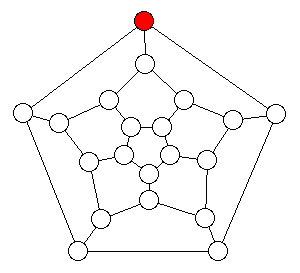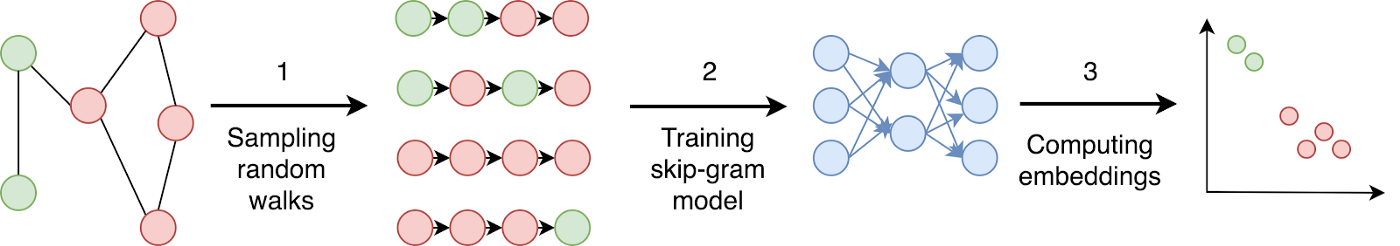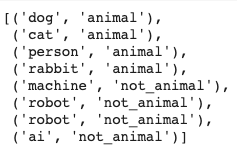# A Gentle Introduction to Embedding Trees and Graphs (with code)Mohammed Terry-Jack

April 7, 2020

Deep Neural Networks (Deep Learning) are dominating advances in Machine Learning and leading to incredible leaps in various fields. However, as prominent figures in the likes of

Gary Marcus and David Cox suggest, there are serious limitations with these approaches. We, too, believe that new approaches are needed, which may even originate in symbolic AI.

This blog post is a primer on how to leverage structured knowledge, i.e. trees and graphs, with deep learning for NLP.

### Contents

2. Example Embedding Methods
3. Embedding WordNet
4. Using the Embedded WordNet in a Downstream ML task
5. Conclusion and code

### 1. Advantages of Tree / Graph Embeddings

Symbolic representations like trees and graphs are favourable due to their interpretable nature, however, they can become computationally burdensome when scaled up and it becomes important to perform searches and other tree/graph-based computations over approximated vector representations of the data structure.

Furthermore, if we wish to utilise structured information from trees and graphs in downstream machine learning tasks (i.e. to recommend new friendships in social networks or predict new drug designs, etc)…

…we must be able to convert the tree or graph into an appropriate representation required by the machine learning algorithm (usually a factored input representation). Therefore we also need embedding methods to encode trees and graphs into compatible vectors.

### Encoding Nodes using Edges

Starting with a very simple method to encode a graph into a vector, each node (or vertex) in the node can be encoded according to the edges which are connected to it.

<pre> def create_vectors_from_edges(graph:Graph) -> List[List[int]]:
return [
[
int(node in edge) for edge in graph.edges
]  for node in graph.nodes
]
</pre>

Unfortunately, the number of edges in a graph can become very large (depending on the complexity of the graph) and so this approach does not scale well.

### Encoding Nodes using Adjacent Nodes

A similar approach is to make each feature of the vector correspond to the nodes themselves (as opposed to the edges). Each node can then be vectorised according to its adjacent / neighbouring nodes.

set(graph[node_id].keys()) | {node_id} for node_id in graph.nodes
]
return list(
map(
lambda neighbours: one_hot_encoding(
indexes=neighbours,
vector_length=len(graph.nodes)
),
)
)
</pre>

Notice how nodes which share similar neighbours are given similar embeddings and thus are plotted close together (indicating they are very similar). The intuition behind why this works becomes clearer if we imagine the nodes represent academic papers (connected in a network of references). Two papers that cite very similar references are assumed to have similar content. Another analogy would be that people with a similar social network would be more similar in personality (or as they say — your friends are a reflection of yourself).

Unfortunately this method only captures local structures formed by immediate neighbours and ignores more global, indirect connections. Embedding methods face an unusual challenge since they must successful encode local neighbourhood relations of each node (i.e. sub-graph structures) while simultaneously maintaining global network information (i.e. topology).

### Encoding Nodes using every Node

This next technique assigns vector features to nodes (again), but instead of only encoding the neighbouring / adjacent nodes, the distance to all other nodes are used as feature values to ensure both local and global structures are encoded in the vector.

<pre> def create_vectors_from_all_nodes(graph:Graph) -> List[List[int]]:
return [
[
sigmoid(shortest_path_length(graph, node_start, node_end)) for node_end in graph.nodes
] for node_start in graph.nodes
]
</pre>

### Encoding Nodes using Parent Nodes

With trees or hierarchical graphs (e.g. WordNet), an special embedding method can make use of the node’s parent nodes to create an embedding (i.e. all the nodes between it and the root node). Unlike using adjacent / neighbouring nodes, this method captures both local and global relational structures. The intuition behind this approach is that nodes with similar ancestral chains will be highly related and thus very similar. For example, if we assume that node 0 is the root node in the graph below, then the vector of node 3 [1,1,1,0,0,0,0] and node 7 [1,1,1,0,0,0,0] are identical since they both share an identical chain of parent nodes (node0, node1, node2).

If you wish, you can also include the current node in the embedding itself to make each embedding slightly unique (e.g. the embedding for node 3 [1,1,1,1,0,0,0] would slightly differ now from its sister node 7 [1,1,1,0,0,0,1]).

<pre> def get_all_parent_nodes(graph:Graph,node_start:int, node_end:int, include_node_end:bool) -> Set[int]:
parent_nodes = reduce(
lambda path_a,path_b: set(path_a)|set(path_b),
all_shortest_paths(graph,node_start,node_end)
)
return parent_nodes if include_node_end else set(parent_nodes) ^ {node_end}
</pre>

We can modify this methods slightly to apply it to a real-world graph known as WordNet:

<pre> def get_all_parent_nodes_wordnet(start_synset, include_node_end:bool) -> list:
parent_nodes = list(
start_synset.closure(lambda synset: synset.hypernyms())
)
return [start_synset] + parent_nodes if include_node_end else parent_nodes
</pre>

However, what you will find when trying to embed the whole of WordNet (or any other large graph) will lead to massive vectors — revealing the problem with some of the techniques described above (i.e. they are limited by the size of the graph since the vector length / number of features is equivalent to the number of nodes in the graph and thus will grow as the network grows — which is not too useful for large or dynamically changing graphs).

### Encoding Nodes using Graph Features

This method equates the vector features to graph features, such as circuits (sub-graphs). The below graph has three sub-graphs and so the resulting vector length can be as small as three values

There are a number of ways to detect these graph features automatically to make this approach scalable.

### Encoding using Artificial Neural Networks

A common approach is to use artificial neural networks to automatically identify sub-graph features within their hidden layers (and hence they will not be interpretable). One of the simplest examples is DeepWalk, which is essentially Word2Vec for graphs (since sentences are like graphs of words).

<pre> from gensim.models import Word2Vecdef create_vectors_from_random_walks(graph:Graph) -> List[List[float]]:
skipgram_model = Word2Vec()
training_set_for_graph = generate_training_set(graph,30,40)
skipgram_model.build_vocab(training_set_for_graph, progress_per=2)
skipgram_model.train(
training_set_for_graph,
total_examples = skipgram_model.corpus_count,
epochs=20,
report_delay=1
)
return skipgram_model[list(map(str,graph.nodes))]
</pre>

To train a skipgram neural network model which underlies word2vec (and thus deepwalk), we need to generate some training ‘sentences’. This is done by creating paths (which are like sentences) from each node (like a seed word). Now we could take every possible path from every node! However, random sampling works just as well.For each node 30 random paths are generated (to ensure a good range of variant paths are captured) using a random walk with a depth of 40 steps (The deeper the walk, the more chance of capturing less localised features).

<pre> from random import choicedef random_walk(node:int, steps:int, graph:Graph) -> List[str]:
path = []
for _ in range(steps):
path.append(str(node))
return path
</pre>

<pre> def generate_training_set(graph:Graph, random_walk_repeats:int, random_walk_path_length:int) -> List[List[str]]:
return [
random_walk(
node = node,
steps = random_walk_path_length,
graph = graph
) for node in graph.nodes for _ in range(random_walk_repeats)
]
</pre>Once the training sentences are generated, the skipgram model is trained and the embeddings obtained from its hidden layer.

You may have noticed from the random walks that the same node is often visited multiple times and that the random exploratory nature of the search is not too effective at building an effective picture of the graph. Node2Vec slightly modifies the random-walk using weights to better control the depth-first / breadth-first aspect of the search / walk.

<pre> from node2vec import Node2Vecdef create_vectors_node2vec(graph:Graph) -> List[List[float]]:
node2vec = Node2Vec(graph, dimensions=64, walk_length=30, num_walks=200, workers=4)
model = node2vec.fit(window=10, min_count=1, batch_words=4)
return model[list(map(str,graph.nodes))]
</pre>

You may have noticed that the approximated feature vectors produced by hidden layers in approaches like deepwalk , node2vec , etc, do not produce as accurate embeddings as the simpler, more exhaustive methods shown earlier. This is true, however, the slight trade-off in accuracy allows for fixed-size vectors that are invariant to the size or complexity of the graph being embedded. Thus the approach can scale to almost any graph.

### Embedding WordNet

Let’s demonstrate how to embed a real-world graph — WordNet — (using the deepwalk method shown above):

<pre> import nltk
from nltk.corpus import wordnetdef get_all_related_synsets(synset) -> list:
return synset.hypernyms() + synset.hyponyms() + synset.part_meronyms() + synset.substance_meronyms() + synset.member_meronyms() + synset.part_holonyms() + synset.substance_holonyms() + synset.member_holonyms() + synset.topic_domains() + synset.region_domains() + synset.usage_domains() + synset.entailments() + synset.causes() + synset.also_sees() + synset.verb_groups() + synset.similar_tos()
</pre>

We slightly modified our previous random walk function to make it compatible with the wordnet graph.

<pre> def random_walk_wordnet(synset,steps:int) -> List[str]:
path = []
for _ in range(steps):
path.append(synset.name())
else:
break
return path
</pre>

Now we are ready to generate our training data from wordnet to train a skipgram model as before.

<pre> def generate_training_set_wordnet(random_walk_repeats:int, random_walk_path_length:int) -> List[List[str]]:
return [
random_walk_wordnet(
synset = synset,
steps = random_walk_path_length,
) for synset in wordnet.all_synsets() for _ in range(random_walk_repeats)
]
</pre>

We then train the skipgram model as before and extract the hidden layers:

And et Voila — we now have WordNet vectors.

### Using the Embedded WordNet in a Downstream Machine Learning task

We can now use these vectors as inputs to a wide range of probabilistic machine learning models (which require factorised representations as inputs). To demonstrate this we train a binary classifier (Support Vector Machine) to identify a word as an “animal” or “not_animal”.We use as few as 8 training examples for our “animal word classifier” (just to give you an idea).

<pre> from sklearn.svm import SVC
svm_classifier = SVC()
svm_classifier.fit(training_inputs, training_output_labels)
</pre>

### Conclusion

In this blog post, our aim was to give an overview to some embedding principals for structured knowledge representations for use in combination with deep learning algorithms. The need for such neural-symbolic AI hybrids stem from deeper limitations of neural networks alone, e.g. its relatively easy to do common-sense reasoning on a graph, but less-so for a neural network.

Structured (and interpretable) knowledge representations (as well as reasoning) are the frontiers of current AI systems. We believe that Neuralsymbolic AI is a way forward that will enable and empower the next generation of AI, where we agree with David Cox from IBM Research on his Lecture on Neuralsymbolic AI at MIT (co-organised by Ava Soleimany) about the potential prospects…

### Try it for yourself

You can find code snippets for the above in the “graph-embeddings” repo on our Github page.

### What’s Next

Join us next time as we look at some of the more sophisticated approaches for embedding graphs, such as Graph Neural Networks and Graph Convolutional Networks.

## Featured